Sun, Dec 10, 2023 @ 11:08 GMT

Leonardo Fibonacci, an Italian mathematician from Pisa, is credited with introducing the Hindu-Arabic numeral system to Europe during the Middle Ages. In his book, Liber Abaci or ‘Book of Calculation’, he also introduced an influential sequence of figures which have come to be known as the Fibonacci numbers.

The relationship between the numbers in this sequence (i.e. the ratio) is not just interesting on a theoretical level. It appears frequently around us in the physical world and is integral for maintaining balance in nature and architecture. It is also important in the financial markets; many traders use Fibonacci ratios to calculate support and resistance levels in their forex trading strategies.

What is the Fibonacci sequence?

Each number in the Fibonacci sequence is calculated by adding together the two previous numbers.

 1 1 2 3 5 8 13 21 34 55 89 144 233 377 … and so on to infinity

What is significant about this pattern, however, is that the ratio of any number to the next one in the sequence tends to be 0.618.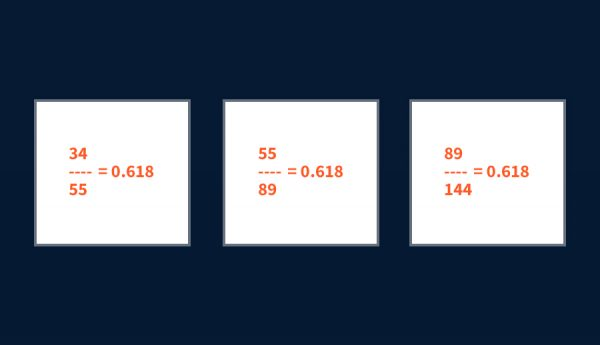Furthermore, the ratio of any number to the number two places ahead in the sequence is always 0.382.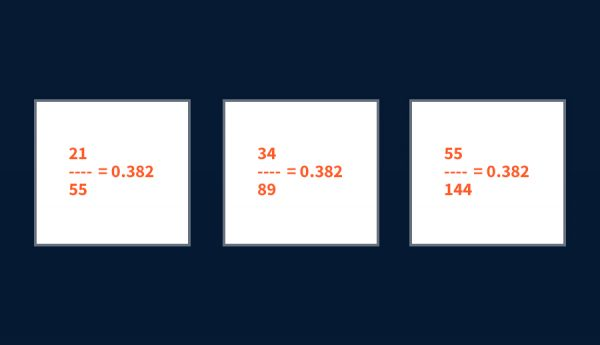Similarly, the ratio of any number to the number three places ahead tends to be 0.236.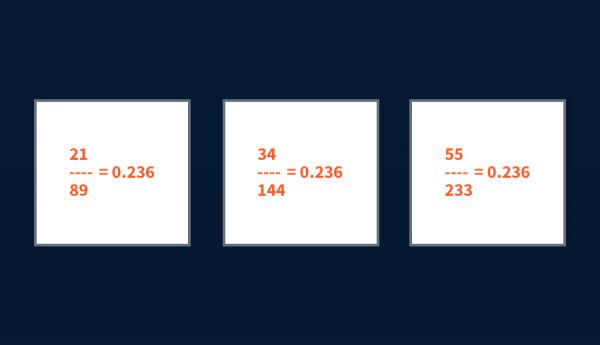These ratios are commonly known as Fibonacci ratios.

Dividing these Fibonacci ratios will result in either 0.618 or 0.382: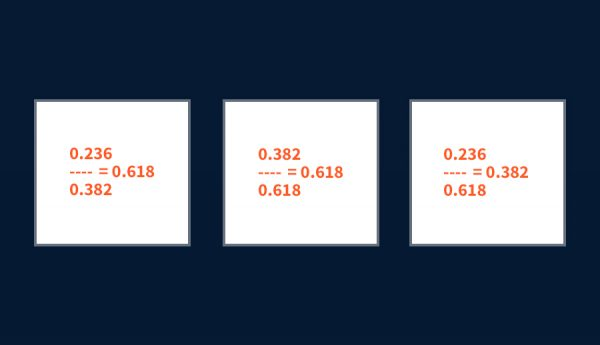### How Fibonacci retracement works

In trading, these ratios are also known as retracement levels. Traders wait for prices to approach these Fibonacci levels and act according to their strategy. Usually, they look for a reversal signal on these widely watched retracement levels before opening their positions. The most commonly used of the three levels is the 0.618 – the inverse of the golden ratio (1.618), denoted in mathematics by the Greek letter φ.

### How to draw Fibonacci retracement levels

Drawing Fibonacci retracement levels is a simple three-step process:

### In an uptrend:

• Step 1 – Identify the direction of the market: uptrend
• Step 2 – Attach the Fibonacci retracement tool on the bottom and drag it to the right, all the way to the top
• Step 3 – Monitor the three potential support levels: 0.236, 0.382 and 0.618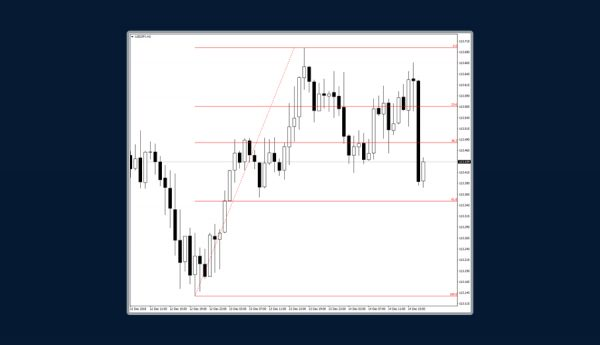### In a downtrend:

• Step 1 – Identify the direction of the market: downtrend
• Step 2 – Attach the Fibonacci retracement tool on the top and drag it to the right, all the way to the bottom
• Step 3 – Monitor the three potential resistance levels: 0.236, 0.382 and 0.618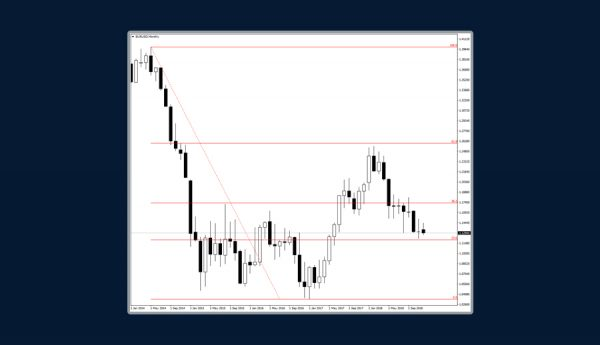Of course, it is more reliable to look for a confluence of signals (i.e. more reasons to take action on a position). Don’t fall into the trap of assuming that just because the price reached a Fibonacci level the market will automatically reverse.

Combine Fibonacci levels with Japanese Candlestick patterns, Oscillators and Indicators for a stronger signal. As you can see in the chart below, the “Three White Soldiers” pattern is confirmed by the fact that prices are trading above the Moving Average line, and additionally that the MACD (Moving Average/Convergence Divergence) is above the zero line.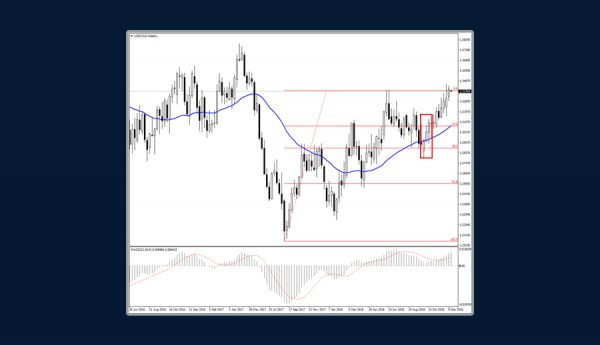Every trader, especially beginners, dreams of mastering the Fibonacci theory. A lot of traders use it to identify potential support and resistance levels on a price chart which suggests reversal is likely. Many enter the market just because the price has reached one of the Fibonacci ratios on the chart. That is not enough! It is better to look for more signals before entering the market, such as reversal Japanese Candlestick formations or Oscillators crossing the base line or even a Moving Average confirming your decision.ForexTimehttp://www.forextime.com/